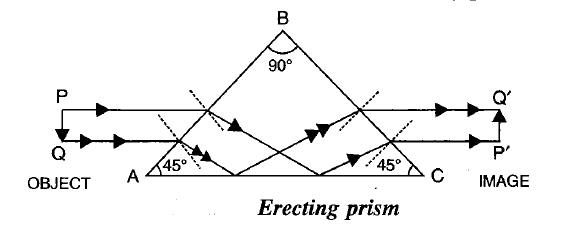# What is a total reflecting prism and when to use it?

Last updated on April 13th, 2021 at 10:23 pm

What is a total reflecting prism? A right-angled isosceles prism that has a 90-degree angle and two 45-degree angles is known as a total reflecting prism.

A total reflecting prism is named so because light ray incident normally on any of its faces suffers total internal reflection inside the prism.

## When to use a total reflecting prism?

Total reflecting prism is used to get (a) 90-degree deviation of light (b) 180-degree deviation of light (c) Inverted image without any deviation of the light ray.

## 1) 90-degree deviation of a ray of light

The total reflecting prism can be used to deviate a light ray by 90 degree when a light ray falls in a specific way on specific side/s of this special type of prism. Visit the link below for a detailed study of that use case.

## 2) 180-degree deviation of a ray of light

The total reflecting prism can deviate a light ray by 180 degrees when a light ray falls in a specific way on a specific side of this special type of prism. Visit the link below for a detailed study of that use case.

## 3) To get an inverted image without causing any deviation of the light rayray diagram of an erecting prism to get an inverted image without causing any deviation of the light ray

A total reflecting prism can also be used to get an inverted image without any deviation of the light ray. Here it can act as an erecting prism. (as shown in the diagram above)

## Conclusion:

From the first and the second cases above, it’s evident that light ray incident normally on any of the faces of the ‘right-angled isosceles prism’ suffers total internal reflection inside the prism. Hence the name of the prism as the total reflecting prism is justified.

See also  Comparing Refraction through a rectangular parallel surfaced glass block and Refraction through a glass prism

## Frequently Asked Questions (answer these after studying the above class notes)

Scroll to top
error: physicsTeacher.in